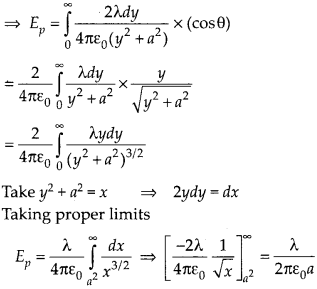Enlightened

# Obtain the formula for the electric field due to a long thin wire of uniform linear charge density X without using Gauss’s law.

• 0

Obtain the formula for the electric field due to a long thin wire of uniform linear charge density X without using Gauss’s law.

Share

1. Solution:
Consider a point P, a unit away from the long charged wire. Electric field due to element dy,vertical components cancel out and horizontal components are added due to symmetryCheck the complete chapter with solutions.

NCERT Solutions for 12th Class Physics: Chapter 1-Electric Charges And Fields

• 0